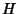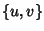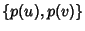## Isomorphic Graphs

Two Graphs which contain the same number of Vertices connected in the same way are said to be isomorphic. Formally, two graphsandwith Verticesare said to be isomorphic if there is a Permutationofsuch thatis in the set of EdgesIffis in the set of Edges.

References

Chartrand, G. ``Isomorphic Graphs.'' §2.2 in Introductory Graph Theory. New York: Dover, pp. 32-40, 1985.